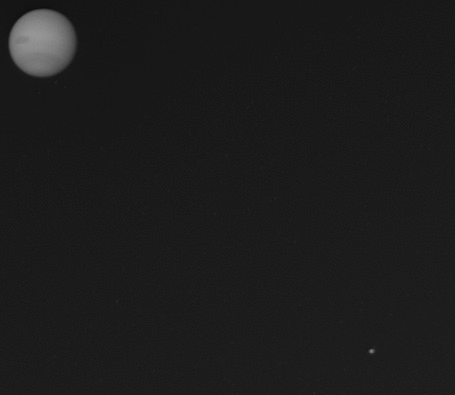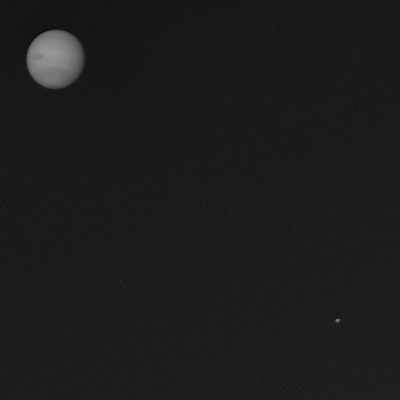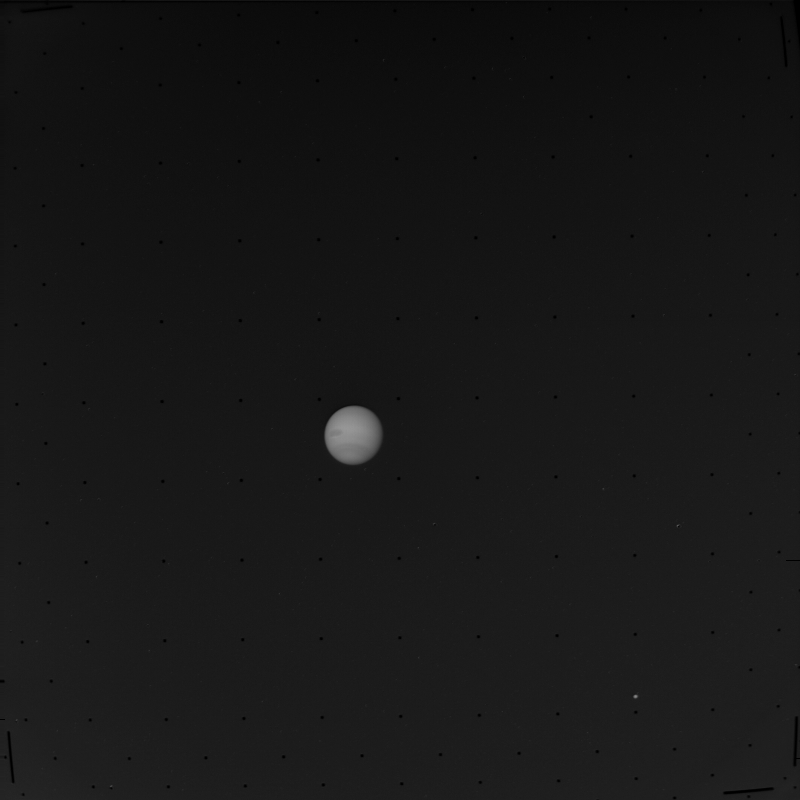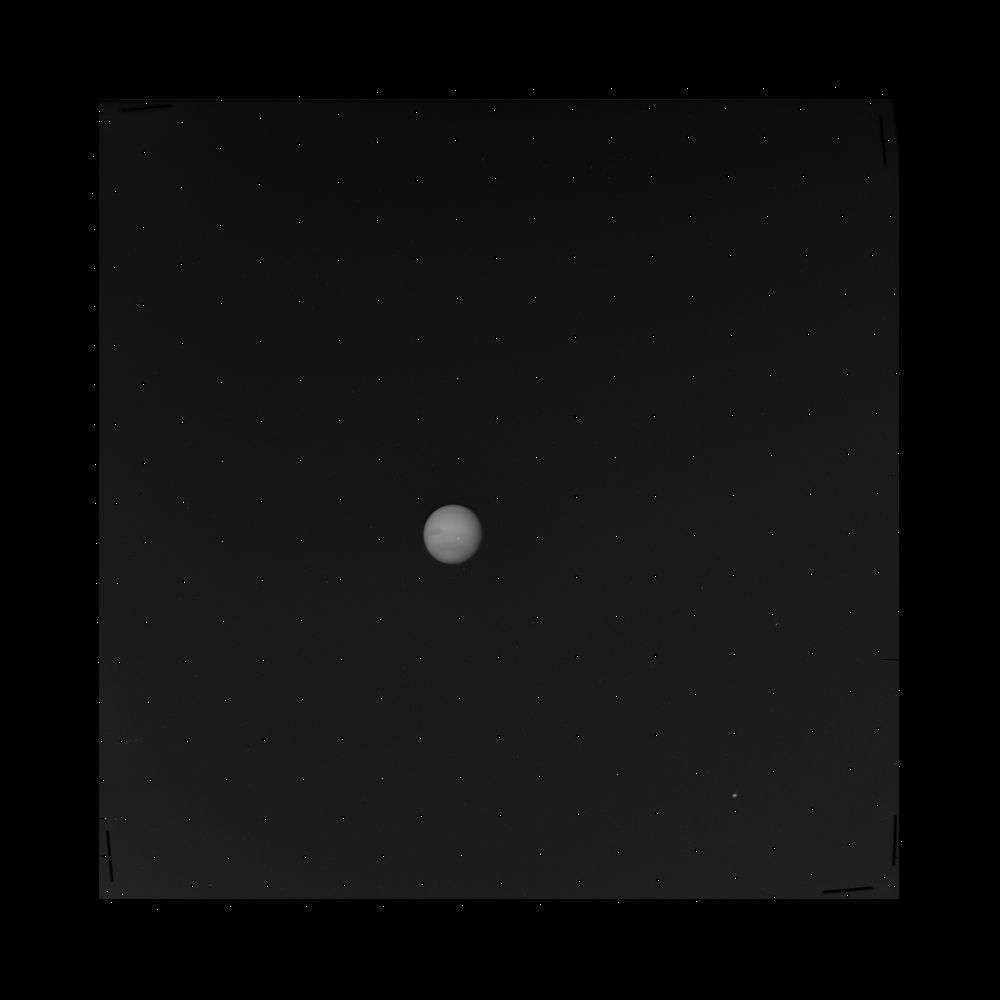Home | Space gifs

# Correcting geometric distortion in Voyager images

I wrote this late January 2013. A month or two later, to my complete astonishment, the geometrically-corrected images became available from the Planetary Data System – people were still updating the official data more than two decades after Voyager 2's Neptune flyby! The following post is therefore unnecessary and probably contains some broken links, but it was a fun exercise and potentially useful for a few weeks.Neptune and Triton, photographed by Voyager 2, 22 June 1989.

In the olden days, NASA used to put vidicon cameras on their spacecraft. For reasons I've never bothered to understand, photographs taken by vidicons can show some geometric distortion.  Triton's orbit looks quite erratic if you use the uncorrected images:For the Voyager flybys of Saturn and Uranus, the NASA/JPL people have corrected the distortions, allowing users to download both the raw and GEOMED* versions of each image (as well as CLEANED and CALIB, two intermediate stages). But for Jupiter and Neptune, any undistorting has to be done ourselves.

*I used GEOMED images for my Saturn's rings post, so that I could fit circles properly.

The engineers knew about the distortion problem, so they put a physical grid of reseau markings in front of the camera. The grid is clear in the raw images:Since the true locations of these reseau markings are known, at a conceptual level the undistortion process is straightforward: locate the reseau points in the raw image, match them up to the true locations, and do a transformation.

Fortunately, we don't have to go and write a program to find the reseau in the raw images: the locations, for both the raw and corrected images, are given in the *_GEOMA.DAT files (in, e.g., this directory). Unfortunately, the numbers are stored in 4-byte VAX floating point format, so I had to go digging through this document to work out how to read them. My resulting Octave code follows; it is quite slow, taking about 15 seconds to extract the points from one _GEOMA.DAT file. Note that Octave stores binary numbers as 1xn arrays.

```bias = 129;

in_file = sprintf("geoma_blog_files\\C0947725_GEOMA.DAT");
out_file = sprintf("geoma_blog_files\\C0947725_GEOMA.csv");

fid_out = fopen(out_file, "w");

fid_in = fopen(in_file, "r");

% The header takes up 1536 bytes:

% Each file contains a 552x4 table, with each "4" comprising 4 bytes,
% so read into a 16x552 matrix.  (More generally you could read the
% table dimensions from the header.)
[geom_array, ct2] = fread(fid_in, [16, 552]);

geom_array = geom_array';

% Initialise a table to hold the reseau locations:
table_array = zeros(552, 4);

for ct_row = 1:552
for ct_col = 1:4
% Split the number into 4 bytes, following notation from
% http://pds.nasa.gov/documents/sr/AppendixC.pdf C.9

m0 = dec2bin(geom_array(ct_row, 4*(ct_col-1)+1), 8);
e1 = dec2bin(geom_array(ct_row, 4*(ct_col-1)+2), 8);
m2 = dec2bin(geom_array(ct_row, 4*(ct_col-1)+3), 8);
m1 = dec2bin(geom_array(ct_row, 4*(ct_col-1)+4), 8);

mantissa_sign = bin2dec(e1(1));
sign_term = -2*(mantissa_sign-0.5);

exponent_bin = [e1(2), e1(3), e1(4), e1(5), e1(6), e1(7), e1(8), m0(1)];
exponent = bin2dec(exponent_bin) - bias;

mantissa_bin = [m0(2:8), m1(1:8), m2(1:8)];
mantissa = bin2dec(mantissa_bin)/2^23;

table_array(ct_row, ct_col) = sign_term*(1+mantissa)*2^exponent;
end
fprintf(fid_out, "%f, %f, %f, %f\n", table_array(ct_row, 1), table_array(ct_row, 2), table_array(ct_row, 3), table_array(ct_row, 4));
end

fclose(fid_out);```

That generates a CSV file with the reseau locations, in the order {output y, output x, input y, input x}. In the exceedingly unlikely event of someone actually verifying this procedure, note that the CSV file, like the _GEOMA.DAT file, will contain loads of duplicate rows – I have no idea why these duplicates exist.

If we plot the points over the top of the raw image, we get a little surprise (I've put the 800x800 raw image into the middle of a 1000x1000 image, because the raw reseau locations are sometimes outside the range 1-800; also note that the output image is 1000x1000):As well as picking out the reseau marks (to within a pixel), the _GEOMA.DAT file also contains rows of points in between the marks, so that all the points (called "tiepoints") can be thought of as vertices of a triangular lattice.

...Which brings us to the transformation from raw image* to undistorted image, which is based on that triangular lattice. I'll skip over the step of working out which triangle each pixel belongs to – good programmers could probably do it easily, but I found it finicky to deal with the slight irregularities in the lattice, and I don't think the procedure I ended up using would be particularly enlightening for anyone.

*Actually I will be converting the _CLEANED image. The _CLEANED image has the dark reseau marks filled in with the average value of the surrounding pixels. This can sometimes cause smudgy artefacts, but generally looks much nicer than the black dots.

The relevant reference is this documentation file for the GEOMA program (first written in 1970!). The overall procedure is to loop over each pixel in the output (undistorted) file, associating each output pixel with pixel co-ordinates from the input (raw) file. Denoting input pixels with dashed variables and output pixels with undashed variables, we want a transform

x' = ax + by + c,
y' = dx + ey + f,

where the coefficients (a, b, c, d, e, f) will be the same for every output pixel in a given triangle. How do we find the values of those coefficients? Well, we have the input and output vertices of the triangle, denoted (x(j), y(j)) for j = 1, 2, 3. So:

x'(1) = ax(1) + by(1) + c,
x'(2) = ax(2) + by(2) + c,
x'(3) = ax(3) + by(3) + c,

and

y'(1) = dx(1) + ey(1) + f,
y'(2) = dx(2) + ey(2) + f,
y'(3) = dx(3) + ey(3) + f.

That's two sets of three simultaneous in three unknowns. In matrix form,

``` / x'(1) \      / x(1)    y(1)    1 \  / a \
|  x'(2)  |  = |  x(2)    y(2)    1  ||  b  |
\ x'(3) /      \ x(3)    y(3)    1 /  \ c /.```

The equation for the y's has the same 3x3 matrix, which I'll call A, on the right-hand side.

Since the matrix A is only a function of the output lattice points, and since the output lattice is the same for all images (because the physical locations of the points in front of the vidicon don't move), it is computationally efficient to pre-calculate the matrix inverse A-1 for each triangle. Then in the loop over each output pixel, we simply look up which triangle it's in, grab the appropriate A-1, and right-multiply that matrix with the vector of input tiepoints for the triangle (once with the x'(j) values, once with the y'(j) values).

That will give us an input pixel (x', y'), but it'll probably be non-integer. To get a smooth-looking output, we'll need to interpolate the pixel value. The same linked reference from above gives us the bilinear interpolation procedure, saving us the effort of working it out from scratch or Wikipedia. Letting the pixel values be denoted by I, we write

I(x, y) = px' + qy' + rx'y' + s.

Letting x1' = floor(x'), x2' = x1'+1, and similarly for y1' and y2', the coefficients (p, q, r, s) are found from the set of four equations

I(x1', y1') = px1' + qy1' + rx1'y1' + s,
I(x1', y2') = px1' + qy2' + rx1'y2' + s,
I(x2', y1') = px2' + qy1' + rx2'y1' + s,
I(x2', y2') = px2' + qy2' + rx2'y2' + s.

And then we have our pixel value for the output pixel (x, y). Loop over the 1000x1000 pixels in the output image, and we've undistorted one picture!

What follows is my Octave code to perform the geometric corrections. It discards any points that are outside the lattice; this might sometimes mean that a few pixels are lost, and it wouldn't be hard to just use the transformation from the nearest triangle, but I wasn't bothered. There are 506 triangles in the lattice; they're stored, along with the relevant 3x3 matrix inverse, in a CSV file. Each pixel is associated with a triangle, and I've stored those in this PNG file, with the red and green colour values summing to the triangle index. On my computer, it takes about 10 minutes to transform one image. I haven't changed the loop over 38 images that ran last night.

```in_pixel_tris = sprintf("geoma_triangles.png");
in_tris_matrices = sprintf("geoma_tris_matrices.csv");

% Load the PNG which says which triangle each pixel belongs to:
pixel_tris = zeros(1000,1000);
pixel_tris = int32(pixel_tris_temp(:,:,1)) + int32(pixel_tris_temp(:,:,2));

% Load the triangle vertices and the matrix inverse for each triangle:

for ct = 1:38
in_tiepoints = sprintf("%d_GEOMA.csv",ct);
in_file = sprintf("clean%d.png", ct);
out_file = sprintf("geomed%d.png", ct);

% Put the 800x800 image into the middle of a 1000x1000 image so that we don't get problems reading negative tiepoints:
input_img = zeros(1000,1000);
input_img(100:899, 100:899) = orig_img;

% Load the tiepoints.  The first two columns are common to all photos
%(at least from what I've checked in Neptune),the second two columns
% vary with each raw photo:

tiepoints = zeros(552,4);

% First remove duplicate rows of tiepoints
tiepoints(1,:) = tiepoints_temp(1,:);
non_dup_ct = 2;

for ct_row = 2:552
if (tiepoints_temp(ct_row, :) == tiepoints_temp(ct_row-1, :))
% Do nothing
else
tiepoints(non_dup_ct, :) = tiepoints_temp(ct_row,:);
non_dup_ct = non_dup_ct + 1;
end
end

num_tiepoints = non_dup_ct - 1;
tiepoints = tiepoints(1:num_tiepoints,:);

tie_out_y = tiepoints(:,1);
tie_out_x = tiepoints(:,2);
% Add 100 pixels to the input tiepoints because we put the image into the middle of a 1000x1000 image:
tie_in_y = tiepoints(:,3) + 100;
tie_in_x = tiepoints(:,4) + 100;

% Initialise the output image:
output_img = zeros(1000,1000);

% Loop over each pixel
for ct_x = 1:1000
for ct_y = 1:1000
% Read the triangle that this pixel is in:
this_tri = pixel_tris(ct_y, ct_x);

% Only do the transformation if we're in a triangle:
if (this_tri > 0)
LHS_matrix_inv = reshape(tris_matrices(this_tri,5:13),3,3);
RHS_x = [tie_in_x(tris_matrices(this_tri,2)); tie_in_x(tris_matrices(this_tri,3)); tie_in_x(tris_matrices(this_tri,4))];
RHS_y = [tie_in_y(tris_matrices(this_tri,2)); tie_in_y(tris_matrices(this_tri,3)); tie_in_y(tris_matrices(this_tri,4))];

% Solve for the transformation coefficients:
params_x = LHS_matrix_inv * RHS_x;
params_y = LHS_matrix_inv * RHS_y;

% Calculate the input pixel corresponding to this output pixel:
in_x = params_x(1)*ct_x + params_x(2)*ct_y + params_x(3);
in_y = params_y(1)*ct_x + params_y(2)*ct_y + params_y(3);

% Need to interpolate pixel value:
in_x1 = floor(in_x);
in_x2 = in_x1+1;
in_y1 = floor(in_y);
in_y2 = in_y1+1;

interp_LHS_matrix = [in_x1, in_y1, in_x1*in_y1, 1; in_x1, in_y2, in_x1*in_y2, 1; in_x2, in_y1, in_x2*in_y1, 1; in_x2, in_y2, in_x2*in_y2, 1];
interp_RHS_vector = [input_img(in_y1, in_x1); input_img(in_y2, in_x1); input_img(in_y1, in_x2); input_img(in_y2, in_x2)];

interp_coeff_vec = interp_LHS_matrix \ interp_RHS_vector;

output_img(ct_y, ct_x) = interp_coeff_vec(1)*in_x + interp_coeff_vec(2)*in_y + interp_coeff_vec(3)*in_x*in_y + interp_coeff_vec(4);
end
end
end

% There shouldn't be any values greater than 255, but just in case....
output_img(output_img>255) = 255;

% Write the undistorted image file:
imwrite(double(output_img)/255, out_file);
end```

Then crop the frames to hold Neptune stationary, adjust the brightness, and we're done!

Home | Space gifs